# JP6107011B2 - Power supply apparatus, voltage output control method, and image forming apparatus provided with power supply apparatus - Google Patents

Power supply apparatus, voltage output control method, and image forming apparatus provided with power supply apparatus Download PDF

## Info

Publication number
JP6107011B2
JP6107011B2 JP2012198830A JP2012198830A JP6107011B2 JP 6107011 B2 JP6107011 B2 JP 6107011B2 JP 2012198830 A JP2012198830 A JP 2012198830A JP 2012198830 A JP2012198830 A JP 2012198830A JP 6107011 B2 JP6107011 B2 JP 6107011B2
Authority
JP
Japan
Prior art keywords
voltage
transformer
output
power supply
rectangular wave
Prior art date
Legal status (The legal status is an assumption and is not a legal conclusion. Google has not performed a legal analysis and makes no representation as to the accuracy of the status listed.)
Active
Application number
JP2012198830A
Other languages
Japanese (ja)
Other versions
JP2014054149A (en
Inventor

Original Assignee

Priority date (The priority date is an assumption and is not a legal conclusion. Google has not performed a legal analysis and makes no representation as to the accuracy of the date listed.)
Filing date
Publication date
Application filed by 株式会社リコー filed Critical 株式会社リコー
Priority to JP2012198830A priority Critical patent/JP6107011B2/en
Publication of JP2014054149A publication Critical patent/JP2014054149A/en
Application granted granted Critical
Publication of JP6107011B2 publication Critical patent/JP6107011B2/en
Active legal-status Critical Current
Anticipated expiration legal-status Critical

• 230000001276 controlling effects Effects 0.000 claims description 4
• 238000000034 methods Methods 0.000 description 9
• 238000004140 cleaning Methods 0.000 description 5
• 230000000694 effects Effects 0.000 description 3
• 230000003247 decreasing Effects 0.000 description 2
• 238000005516 engineering processes Methods 0.000 description 1

## Images

•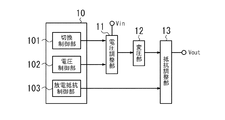•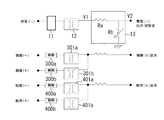•••••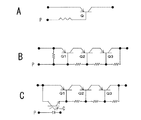••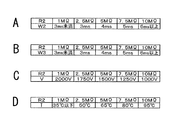••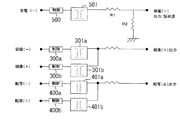•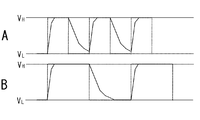•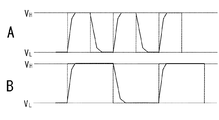## Description

The present invention relates to a power supply device that alternately outputs a high voltage and a low voltage, a voltage output control method, and an image forming apparatus including the power supply device.

2. Description of the Related Art Conventionally, electrophotographic image forming processing has been performed in image forming apparatuses such as copying machines, facsimile machines, and printers. In the electrophotographic image forming process, an electrostatic latent image is formed on a photoreceptor, the formed electrostatic latent image is developed with toner, and the developed toner image is transferred to a recording medium to form an image. . In such an electrophotographic process, an AC voltage waveform may be used during toner charging during the development process and cleaning process of the photoreceptor.
In the cleaning process of the photosensitive member, the cleaning effect depends on the frequency of the AC voltage waveform, and is proportional to the image forming processing speed (= the linear speed of the photosensitive member). An AC power transformer can be used to generate such an AC voltage waveform. However, since an AC power transformer is expensive and large in size, it is small and low-priced from the viewpoint of cost reduction and miniaturization. There has been proposed a method of generating a rectangular waveform by alternately outputting DC voltage waveforms having different voltage values using a voltage power source to form a pseudo AC voltage waveform.

For example, Patent Document 1 describes a method using two DC voltage power supplies that output different voltage values in order to generate a rectangular wave. Further, in Patent Document 2, a first dynamic range for high voltage adjustment and a second dynamic range for low voltage adjustment in which the rate of change of the output voltage with respect to the duty ratio is smaller than the first dynamic range for high voltage adjustment. The output voltage is adjusted by adjusting the duty ratio of the high-voltage PWM (Pulse Width Modulation) signal using the first dynamic range when adjusting the high-voltage output, and the second when adjusting the low-voltage output. It is described that by operating the duty ratio of the low-voltage PWM signal using the dynamic range, a voltage value output from one power transformer is changed to output a rectangular wave in which different voltage values are combined.

FIG. 10 is a schematic configuration diagram relating to a conventional power supply device of an image forming apparatus. In this example, a power supply device that supplies a high voltage to the charging unit, the developing unit, and the transfer unit is shown.
In order to supply high-voltage power in the charging unit, two voltage control units 200a and 200b and a transformer unit 201a and 201b including a power transformer are connected to output a high voltage from the transformer unit 201a and a low voltage from the transformer unit 201b. Is output. The voltage control units 200a and 200b are configured to output a rectangular negative voltage by alternately combining high voltage and low voltage. A voltage dividing resistor R1 and a discharge resistor R2 are connected to the output side of the transformers 201a and 201b, and these are applied to the discharge action on the high voltage side that occurs when the generated rectangular wave falls from the high voltage to the low voltage. Resistance is involved.

Further, in order to supply high voltage power in the developing unit, two voltage control units 300a and 300b and a transformer unit 301a and 301b including a power transformer are connected, and a negative voltage is output from the transformer unit 301a and the transformer unit. A positive voltage is output from 301b. When the developing process is performed on the photosensitive member, the voltage control unit 300a controls the transformation unit 301a to output a negative voltage. When the cleaning process is performed, the voltage control unit 300b causes the transformation unit 301b to It is controlled so that a positive voltage is output.
Further, in order to supply high voltage power in the transfer unit, two voltage control units 400a and 400b and a transformer unit 401a and 401b including a power transformer are connected, and a negative voltage is output from the transformer unit 401a and the transformer unit. A positive voltage is output from 401b. When the transfer process is performed on the photosensitive member, the voltage control unit 401a controls the transformation unit 401a to output a negative voltage. When the cleaning process is performed, the voltage control unit 400b causes the transformation unit 401b to It is controlled so that a positive voltage is output.

In the conventional example described above, two power transformers are used as the power unit of the charging unit. Therefore, as described in Patent Document 2, if a rectangular wave is output by one power transformer, two power transformers are used. Cost and space can be saved as compared with a power supply device using a DC voltage power supply. FIG. 11 is a schematic configuration diagram relating to a conventional example including a voltage control unit 500 and a transformer unit 501 including one power transformer as a power supply device for a charging unit. In this example, the voltage control unit 500 can alternately generate different voltages of high voltage and low voltage from the transformer unit 501 to obtain a rectangular wave output voltage. The power supply device for the developing unit and the transfer unit is the same as that shown in FIG.
As in the conventional example shown in FIG. 11, in the method of outputting a rectangular wave in which different voltage values are combined by changing the voltage value using a single power transformer, the rise time and the fall time of the output waveform of the rectangular wave However, since the rise time depends on the capability of the power transformer, it can be set to rise in a relatively short time. Further, since the fall time of the rectangular wave waveform depends on the discharge characteristics, it is necessary to set the discharge resistance low in order to shorten the fall time.

FIG. 12 is a waveform diagram of a rectangular wave when the discharge resistance is high (eg, 10 MΩ). FIG. 12A shows a case where the frequency of the rectangular wave is high, and FIG. 12B shows a case where the frequency of the rectangular wave is low. In the waveform diagram, an ideal voltage waveform is indicated by a dotted line, and an actual voltage waveform is indicated by a solid line. The rectangular wave is formed by alternately outputting a high voltage (V H ) and a low voltage (V L ). When the rectangular wave falls from a high voltage to a low voltage, it discharges from the high voltage via the discharge resistance and becomes a low voltage. Therefore, the fall time from the high pressure to the low pressure is determined mainly based on the discharge resistance. When the discharge resistance is high, the fall time becomes long. Therefore, when the frequency of the rectangular wave becomes high, as shown in FIG. It becomes difficult to output.
FIG. 13 is a waveform diagram of a rectangular wave when the discharge resistance is low (eg, 1 MΩ). FIG. 13A shows a case where the frequency of the rectangular wave is high, and FIG. 13B shows a case where the frequency of the rectangular wave is low. As in FIG. 12, the waveform diagram shows an ideal voltage waveform with a dotted line and an actual voltage waveform with a solid line. When the discharge resistance is lowered, the fall time from the high pressure to the low pressure is shortened. Therefore, as shown in FIG. can do.

As described above, when the fall time of the output waveform of the rectangular wave cannot be sufficiently shortened, the voltage rises without sufficiently decreasing at the time of falling at a high frequency, so that the image forming processing speed of the image forming apparatus is increased. When it becomes larger, it becomes impossible to output a high-frequency rectangular wave corresponding to it. However, if the discharge resistance value is set low as shown in FIG. 13 in order to improve the discharge characteristics, current is consumed by the discharge resistance even during output. For this reason, it is necessary to increase the output of the power transformer and the capacity of the drive circuit in accordance with the decrease in the discharge resistance value, thereby reducing the cost and space saving effect and eliminating the merit of reducing the power transformer to one. was there.

Therefore, the present invention has been made in view of such problems of the conventional technology, and an object thereof is to perform stable rectangular wave output by changing the discharge resistance in accordance with the frequency of the rectangular wave output voltage.

A power supply device according to the present invention includes: a voltage transformation unit including a single power transformer; a voltage adjustment unit that is connected to an input side of the voltage transformation unit and outputs a plurality of different voltage values from the voltage transformation unit; and the voltage adjustment unit. A control unit that controls to output a rectangular wave voltage from the transformer unit, and is connected to an output side of the transformer unit, and adjusts a discharge resistance according to the frequency of the rectangular wave to adjust the high voltage of the rectangular wave It is a power supply device provided with the resistance adjustment part which adjusts the fall time to low pressure from.

According to the present invention, depending on the frequency of the square wave, since by adjusting the discharge resistance from the high pressure of the rectangular wave can be adjusted fall time to low pressure, it is possible to perform the rectangular wave output was cheap boss .

It is a schematic block diagram regarding embodiment which concerns on this invention. It is a schematic block diagram regarding the example which used the power supply device shown in FIG. 1 for the image forming apparatus. It is a table | surface which shows the example of a setting of the output voltage at the time of changing a variable resistance. FIG. 3 is a schematic configuration diagram relating to a modified example of the image forming apparatus illustrated in FIG. 2. It is a table | surface which shows the example of a setting of the output voltage at the time of changing the variable resistance for voltage division according to the change of the variable resistance used as discharge resistance. It is a circuit diagram which shows the structural example of the variable resistance which changes discharge resistance in a resistance adjustment part. It is a circuit diagram which shows the structural example of the variable resistance which changes discharge resistance in a resistance adjustment part. It is explanatory drawing regarding the specific example of a setting in the case of adjusting discharge resistance. It is explanatory drawing regarding the specific example of a setting in the case of adjusting discharge resistance. It is a schematic block diagram regarding the conventional power supply device of an image forming apparatus. It is a schematic block diagram regarding the prior art example provided with the voltage control part and the transformation part which consists of one power transformer as a power supply device of a charging part. It is a waveform diagram of a rectangular wave when the discharge resistance is high. It is a waveform diagram of a rectangular wave when the discharge resistance is low.

FIG. 1 is a schematic configuration diagram relating to an embodiment of the present invention. The power supply device includes a control unit 10, a voltage adjustment unit 11, a voltage transformation unit 12, and a resistance adjustment unit 13. The control unit 10 performs processing for performing voltage output control, a switching control unit 101 that performs high-voltage and low-voltage switching processing of the output voltage, and voltage control that performs processing so that high-voltage and low-voltage voltages are output from the transformer 12. Unit 102 and a discharge resistance control unit 103 that adjusts the discharge resistance of the voltage output from the transformer 12.
The switching control unit 101 performs switching processing by performing output control of a switching signal based on a preset period of a rectangular wave. As the switching signal, for example, a signal obtained by alternately combining a high level and a low level corresponding to the high voltage and low voltage of the output voltage is output and transmitted to the voltage adjustment unit 11, as described later. The voltage of the rectangular wave that is alternately combined can be output from the transformer 12.

The voltage control unit 102 outputs a voltage control signal to the voltage adjustment unit 11 and controls the voltage adjustment unit 11 to stably output high and low voltage from the transformer 12 based on the input voltage Vin. As the voltage control signal, for example, a PWM signal having a predetermined duty ratio is output to the voltage adjustment unit 11, and the current that flows in the input-side winding of the power transformer of the transformer 12 is controlled in the voltage adjustment unit 11. As will be described later, when the output voltage Vout changes when the discharge resistance on the output side of the transformer 12 is changed, voltage control is performed so that the output voltage Vout becomes constant.
The discharge resistance control unit 103 outputs a resistance control signal to the resistance adjustment unit 13 that adjusts the discharge resistance of the voltage Vout output from the transformer 12. The resistance control signal is output so as to adjust the discharge resistance in order to adjust the fall time of the rectangular wave from the high voltage to the low voltage. For example, when the frequency becomes equal to or higher than a predetermined frequency, output is performed so as to decrease the discharge resistance, or output so that the discharge resistance is variable according to the frequency. Since the frequency of the rectangular wave is set when the switching signal is output from the switching control unit 101, the resistance control signal can be output according to the frequency of the switching signal. In addition, when adjusting the falling time of the rectangular wave from high voltage to low voltage, in addition to the frequency of the rectangular wave, the voltage difference between the rectangular wave low voltage section or high voltage section, the rectangular wave high voltage and low voltage The resistance control signal can also be output according to parameters such as the temperature of the drive circuit element in the power supply device.

The voltage adjustment unit 11 adjusts the output voltage of the transformer 12 based on the switching signal from the switching control unit 101 and the voltage control signal from the voltage control unit 102. The transformer 12 includes a single power transformer, and the voltage regulator 11 divides the input voltage Vin based on a switching signal transmitted from the switching controller 101, so that the input of the power transformer of the transformer 12 The side voltage is switched to high voltage or low voltage, and the output side voltage of the power transformer is output as a rectangular wave voltage in which high voltage or low voltage is alternately combined. Further, when a PWM signal is input as a voltage control signal from the voltage control unit 102, the current flowing in the input side winding of the power transformer of the transformer 12 is controlled according to the duty ratio of the PWM signal, and the transformer 12 Is adjusted so as to stably output a high or low output voltage.
The transformer 12 includes a single power transformer, and a current is passed through the input winding of the power transformer based on the voltage control signal from the voltage regulator 11, so that the input side voltage is applied to the output winding. On the other hand, an output voltage with a predetermined ratio is generated.

The resistance adjuster 13 is connected to the output side of the transformer 12 and adjusts the discharge resistance based on a resistance control signal from the discharge resistance controller 103. By adjusting the discharge resistance, the falling time from the high voltage to the low voltage of the rectangular wave output from the transformer 12 is adjusted so that the high voltage and the low voltage of the rectangular wave can be changed stably. Since the fall time is shortened by lowering the discharge resistance, the discharge resistance is adjusted so as to be lowered when the frequency of the rectangular wave is increased, so that the rectangular wave is surely changed from high pressure to low pressure. The discharge resistance can be adjusted, for example, by connecting a variable resistor to the output side of the transformer 12 and changing the resistance of the variable resistor. Further, a transistor can be connected to the output side of the transformer 12 and the base current can be changed to control the current between the collector and the emitter to change the apparent resistance. If the output voltage changes by adjusting the discharge resistance with a variable resistor, connect the variable resistor for voltage division to the variable resistor and adjust the discharge resistance so that the voltage division ratio is constant. The voltage dividing resistance can be adjusted in accordance with the above.

When a rectangular wave output voltage is obtained, the allowable time required to reach the predetermined voltage value varies depending on the frequency because the voltage reciprocates between the high voltage and the low voltage. The minimum required rise time and fall time vary depending on the frequency, and the minimum required rise time and fall time are shorter when the frequency is higher than when the frequency is low. When outputting with a rectangular wave, the fall time from high pressure to low pressure is governed by the discharge action, and apparently the output of high pressure is stopped, so continuous operation with high pressure is higher when the frequency is higher than when the frequency is low. As time is reduced, the power transformer and drive circuit can withstand higher power. Also, the fall time of the rectangular wave from the high pressure to the low pressure is governed by the discharge time until it reaches the low pressure, so the fall time can be shortened if the discharge resistance value is set low. Further, since the output from the transformer is consumed even in the discharge resistance portion, it is necessary to increase the output if the discharge resistance value is set low.
Considering the above points, when a rectangular wave output voltage is obtained by alternately outputting a high voltage and a low voltage by a single power transformer, it is desirable to set the discharge resistance value lower as the frequency of the rectangular wave increases. . Therefore, by detecting the frequency of the rectangular wave and making the discharge resistance variable according to the frequency, the range of frequencies that can be output can be expanded.

The frequency of the rectangular wave can be detected by measuring the period of the output voltage. Further, since the cycle of the switching signal output from the switching control unit has a correlation with the cycle of the output voltage, the discharge resistance can be adjusted based on the cycle of the switching signal. Further, in order to adjust the fall time of the rectangular wave from the high voltage to the low voltage, the discharge resistance can be adjusted based on the output time of the portion corresponding to the low voltage section of the switching signal.
When the discharge resistance value is changed based on the frequency thus detected, the discharge resistance can be changed in accordance with the frequency by using a variable resistor such as a “volume resistor” as the discharge resistance. Further, as described above, a transistor can be used as the discharge resistor. By changing the base current of the transistor by the PWM signal, the current between the collector and emitter of the transistor (in the case of an NPN transistor) can be changed, and the apparent resistance value of the transistor can be changed.

FIG. 2 is a schematic configuration diagram regarding an example in which the power supply device shown in FIG. 1 is used in an image forming apparatus. In this example, the power supply device shown in FIG. 1 is used as a high-voltage power supply for the charging unit, and the resistance adjustment unit 13 includes a fixed resistor Ra for voltage division and a variable resistor Rb serving as a discharge resistor. As the variable resistor Rb, for example, a resistor whose resistance can be changed in the range of 1 to 10 MΩ can be used. The high-voltage power supply for the developing unit and the transfer unit is the same as the high-voltage power supply shown in FIG. The voltage adjusting unit 11 can alternately output a high voltage and a low voltage from the transformer 12 to obtain a rectangular wave output voltage V1. The output voltage V1 is input to the resistance adjusting unit 13 to obtain the output voltage V2. By using the variable resistor Rb as the discharge resistor in the resistance adjusting unit 13, the variable resistor Rb is set high in the case of a low frequency in accordance with the frequency of the rectangular wave output voltage V1, and current consumption due to the discharge resistor is suppressed. In the case of a high frequency, the variable resistor Rb is set low, and the fall time from the high voltage to the low voltage due to the discharge resistor is shortened, so that the output voltage V2 can be a stable rectangular wave even at a high frequency.
When the variable resistor Rb is changed, the voltage dividing ratio with the fixed resistor Ra is changed. Therefore, the output voltage V1 is changed according to the set value of the variable resistor Rb so that the output voltage V2 becomes a constant voltage value. Voltage control is performed. FIG. 3 is a table showing a setting example of the output voltage V1 when the variable resistor Rb is changed. In this example, when the fixed resistor Ra is 1000Ω and the variable resistor Rb is changed from 1 MΩ to 10 MΩ, the output voltage V2 is constant at 1000V by controlling the voltage so that the output voltage V1 is decreased from 1100V to 1010V. So that the voltage is controlled.

FIG. 4 is a schematic configuration diagram regarding a modification of the image forming apparatus shown in FIG. In this example, the resistance adjustment unit 13 ′ of the power supply device used as the high-voltage power source of the charging unit includes a variable resistor Ra ′ for voltage division and a variable resistor Rb ′ serving as a discharge resistor. As the variable resistor Ra ′, for example, a resistor whose resistance can be changed in a range of 100Ω to 1000Ω can be used. As the variable resistor Rb ′, a resistor whose resistance can be changed in a range of 1 to 10 MΩ can be used. By setting the variable resistor Rb ′ serving as the discharge resistor low, the fall time of the rectangular wave from the high voltage to the low voltage can be shortened, and the variable resistor Ra ′ when the resistance value of the variable resistor Rb ′ is changed If the variable resistor Ra ′ is changed to maintain a constant ratio, the voltage control can be performed without changing the output voltage V1. FIG. 5 is a table showing a setting example of the output voltage V1 when the voltage dividing variable resistor Ra 'is changed in accordance with the change of the variable resistor Rb' serving as the discharge resistor. In this example, when the variable resistor Rb ′ is changed from 1 MΩ to 10 MΩ, the variable resistor Ra ′ is changed from 100Ω to 1000Ω in accordance with the change, and the output voltage V1 is controlled to be constant at 1010V, so that the output The voltage V2 is stably output at 1000V.

6 and 7 are circuit diagrams illustrating a configuration example of a variable resistor that changes the discharge resistance in the resistance adjusting unit. FIG. 6 shows a case where a volume resistor is used as the variable resistor. The volume resistor can be adjusted to a predetermined resistance value according to the frequency of the output rectangular wave. When adjusting a volume resistor, the method of adjusting manually or the method of adjusting using drive mechanisms, such as a motor, is mentioned. In the case of using a drive mechanism, the volume resistor can be adjusted by controlling the drive with a resistance control signal.
FIG. 7 is a circuit diagram illustrating a configuration example of a variable resistor using a transistor. In FIG. 7A, the transistor Q is connected as a discharge resistor, and a PWM signal P is input as a resistance control signal to the base of the transistor Q to control the base current. By controlling the base current, the apparent resistance can be changed by adjusting the current between the emitter and collector of the transistor (in the case of a PNP transistor). FIG. 7B is a circuit diagram relating to a configuration example in which transistors are connected in a plurality of stages.

In this example, emitters and collectors of a plurality of transistors are connected in series, and a PWM signal P is input as a resistance control signal to the base of each transistor. When the difference between the input voltage and output voltage of a transistor exceeds the breakdown voltage of the transistor, the voltage applied to each individual transistor can be reduced and set within the breakdown voltage range by connecting multiple transistors in series. Can do. In the example shown in FIG. 7B, by connecting the same three transistors Q1 to Q3 in series, the voltage applied to one transistor can be reduced to 1/3, and even a transistor with a low breakdown voltage is used. It becomes possible to do. Further, by increasing the number of transistors connected in series, it is possible to cope with an increase in applied voltage. FIG. 7C has a photocoupler C connected to the base of the transistor in the configuration example shown in FIG. 7B. A PWM signal P is input as a resistance control signal to the photocoupler C, and an output signal of the photocoupler C is input to the base of each transistor. In this example, by adding the photocoupler C, the voltage applied to the transistor is reduced accordingly, the breakdown voltage of the transistor can be improved as a whole, and the discharge resistance can be adjusted stably. . Further, by using a photocoupler, an increase in space and cost can be suppressed as compared with the case where transistors are increased.

8 and 9 are explanatory diagrams relating to specific setting examples when adjusting the discharge resistance. FIG. 8 is a waveform diagram regarding the voltage of the rectangular wave, where the period of the rectangular wave is W1, the time of the low pressure section in one period is W2, and the time of the high voltage section is W3. Further, the voltage difference between the high voltage and the low voltage (the fluctuation width in which the voltage changes) is V. FIG. 9A is a setting table showing the relationship between the discharge resistance R2 and the time W2 of the low pressure section. The discharge resistance R2 is set to be lower as the time W2 of the low pressure section is shorter. Since the fall time from the high pressure to the low pressure is mainly determined by the discharge action, it is necessary to improve the discharge characteristics as the time W2 in the low pressure section is shorter. Therefore, the fall time to a low voltage | pressure can be shortened by making discharge resistance R2 low. In addition, if the time W2 in the low voltage section becomes longer, the time during which the power transformer of the transformer and its drive circuit are not operating can be lengthened, and a larger output becomes possible. Therefore, the discharge resistance R2 is set low and consumed. It can withstand the increase in power.
FIG. 9B is a setting table showing the relationship between the discharge resistance R2 and the time W3 of the high voltage section. The discharge resistance R2 is set to be higher as the time W3 of the high pressure section is longer. If the time W3 of the high voltage section becomes longer, the time during which the power transformer and its drive circuit in the transformer section are continuously operated becomes longer. Therefore, by setting the discharge resistance R2 high, the range of the capability of the power transformer and its drive circuit Can be used within. Note that the same effect as in the case of the time W3 in the high voltage section can also be obtained by setting the discharge resistance R2 according to the period W1 of the rectangular wave.
FIG. 9C is a setting table showing the relationship between the discharge resistor R2 and the voltage difference V. The discharge resistance R2 is set to be lower as the voltage difference V is larger. As the voltage difference V increases, the voltage value when changing from a high voltage to a low voltage increases. When the predetermined fall time is set, the discharge resistance R2 is set low because it is necessary to increase the fall speed when the voltage difference V is larger than when the voltage difference V is small.

FIG. 9D is a setting table showing the relationship between the discharge resistor R2 and the temperature T of the drive circuit element in the power supply device. The higher the temperature T of the drive circuit element, the higher the discharge resistance R2. As the power consumption of the power supply increases, the amount of heat generated by the drive circuit elements in the power supply increases and the temperature rises. In this case, it is necessary to suppress the temperature rise to the heat resistant temperature which is the limit temperature at which the drive circuit element operates normally. As the discharge resistance R2 is larger, the power consumed via the discharge resistance R2 is smaller. Therefore, if the discharge resistance R2 is determined based on the heat-resistant temperature of the drive circuit element, appropriate control can be performed. It is also conceivable that the discharge resistance R2 is set to a predetermined value up to the heat resistant temperature of the drive circuit element in the power supply device, and the discharge resistance R2 is changed to a large value before the heat resistant temperature is exceeded. It is also conceivable to set a temperature table relating to the control element in the power supply device for each use condition of the power supply device, and to create and control a discharge resistance setting table using such a temperature table.

DESCRIPTION OF SYMBOLS 10 ... Control part, 11 ... Voltage adjustment part, 12 ... Transformer part, 13 ... Resistance adjustment part.

JP-A-7-287620 Japanese Patent No. 3743104

## Claims (6)

1. A transformer including a single power transformer, a voltage regulator connected to an input side of the transformer and outputting a plurality of different voltage values from the transformer, and a rectangular from the transformer by controlling the voltage regulator. A control unit for outputting a voltage of the wave and an output side of the transformer , and adjusting a discharge resistance according to the frequency of the rectangular wave to reduce a fall time of the rectangular wave from a high voltage to a low voltage A power supply device comprising a resistance adjustment unit for adjustment.
2.   The power supply device according to claim 1, wherein the resistance adjustment unit adjusts a discharge resistance based on a resistance control signal transmitted from the control unit.
3.   The power supply device according to claim 2, wherein the control unit transmits a resistance control signal so as to adjust a discharge resistance based on a time of a high voltage section or a low pressure section of the rectangular wave.
4.   The power supply device according to claim 2, wherein the control unit transmits a resistance control signal so as to adjust a discharge resistance based on a temperature of a drive circuit element in the device.
5.   An image forming apparatus comprising the power supply device according to claim 1.
6. In a voltage output control method for outputting a rectangular wave voltage in which a high voltage and a low voltage are alternately combined from a single power transformer, the discharge resistance on the output side of the power transformer is adjusted in accordance with the frequency of the rectangular wave, and the rectangular A voltage output control method that adjusts the fall time of a wave from high voltage to low voltage.
JP2012198830A 2012-09-10 2012-09-10 Power supply apparatus, voltage output control method, and image forming apparatus provided with power supply apparatus Active JP6107011B2 (en)

## Priority Applications (1)

Application Number Priority Date Filing Date Title
JP2012198830A JP6107011B2 (en) 2012-09-10 2012-09-10 Power supply apparatus, voltage output control method, and image forming apparatus provided with power supply apparatus

## Applications Claiming Priority (1)

Application Number Priority Date Filing Date Title
JP2012198830A JP6107011B2 (en) 2012-09-10 2012-09-10 Power supply apparatus, voltage output control method, and image forming apparatus provided with power supply apparatus

## Publications (2)

Publication Number Publication Date
JP2014054149A JP2014054149A (en) 2014-03-20
JP6107011B2 true JP6107011B2 (en) 2017-04-05

# Family

## Family Applications (1)

Application Number Title Priority Date Filing Date
JP2012198830A Active JP6107011B2 (en) 2012-09-10 2012-09-10 Power supply apparatus, voltage output control method, and image forming apparatus provided with power supply apparatus

## Country Status (1)

JP (1) JP6107011B2 (en)

## Family Cites Families (4)

* Cited by examiner, † Cited by third party
Publication number Priority date Publication date Assignee Title
JP3247128B2 (en) * 1991-10-09 2002-01-15 富士通株式会社 Variable delay circuit
JP3612932B2 (en) * 1997-04-18 2005-01-26 富士ゼロックス株式会社 High voltage power supply
KR100505645B1 (en) * 2002-10-17 2005-08-03 삼성전자주식회사 Output driver capable of controlling slew rate of output signal according to operating frequency information or CAS latency information
JP4264277B2 (en) * 2003-03-17 2009-05-13 株式会社リコー Constant voltage power supply device, developing device, and image forming apparatus

## Also Published As

Publication number Publication date
JP2014054149A (en) 2014-03-20

## Similar Documents

Publication Publication Date Title
US9337731B2 (en) Power converter for generating both positive and negative output signals
US9362833B2 (en) Constant voltage constant current control circuits and methods with improved load regulation
US7675758B2 (en) Power converter with an adaptive controller and method of operating the same
EP2637295B1 (en) Power supply device and image forming apparatus
US9130468B2 (en) Constant voltage constant current controller and control method thereof
CN101795074B (en) Power supply controller with input voltage compensation for efficiency and maximum power output
TW552769B (en) Circuit arrangement to generate a switch-signal for a current-controlled switching power-supply
US8891268B2 (en) System and method for soft-starting an isolated power supply system
TWI489757B (en) Switching power converter and method for controlling the same
JP5587051B2 (en) Switching power supply
JP5481926B2 (en) Power supply control device, power supply device, and power supply control method
US8295062B2 (en) Switching power supply apparatus and semiconductor device
CN103477548B (en) Switching power source device control circuit and Switching Power Supply
CN101356719B (en) Improving transient behavior while switching between control loops in a switching voltage regulator
US9019729B2 (en) Controller for a switched mode power converter
US6977830B2 (en) Power supply apparatus
JP4468011B2 (en) Switching power supply and image forming apparatus
KR100889528B1 (en) Soft start circuit and power supply including the circuit
US20050046404A1 (en) Controller for complementary switches of a power converter and method of operation thereof
US7663896B2 (en) Energy effective switching power supply apparatus and an energy effective method thereof
JP4156819B2 (en) Method and apparatus for reducing audible noise in switching regulators
US8456870B2 (en) Power supply and image forming device having the same
US20080084198A1 (en) Method and apparatus for a power supply controller responsive to a feedforward signal
KR20120066603A (en) Dc/dc converter, and power supply and electronic device using the same
JP6218467B2 (en) Power supply device and image forming apparatus

## Legal Events

Date Code Title Description
A621 Written request for application examination

Free format text: JAPANESE INTERMEDIATE CODE: A621

Effective date: 20150907

A977 Report on retrieval

Free format text: JAPANESE INTERMEDIATE CODE: A971007

Effective date: 20160630

A131 Notification of reasons for refusal

Free format text: JAPANESE INTERMEDIATE CODE: A131

Effective date: 20160705

A521 Written amendment

Free format text: JAPANESE INTERMEDIATE CODE: A523

Effective date: 20160905

TRDD Decision of grant or rejection written
A01 Written decision to grant a patent or to grant a registration (utility model)

Free format text: JAPANESE INTERMEDIATE CODE: A01

Effective date: 20170207

A61 First payment of annual fees (during grant procedure)

Free format text: JAPANESE INTERMEDIATE CODE: A61

Effective date: 20170220

R151 Written notification of patent or utility model registration

Ref document number: 6107011

Country of ref document: JP

Free format text: JAPANESE INTERMEDIATE CODE: R151